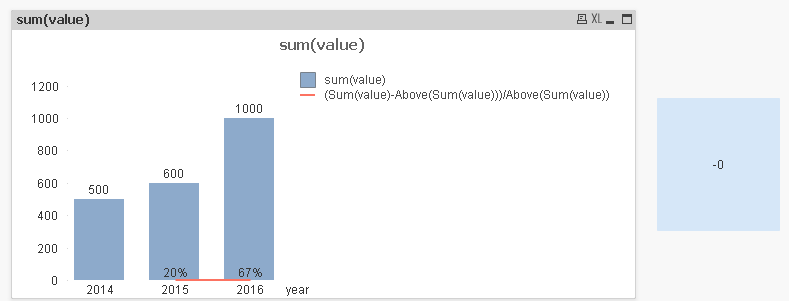# QlikView Creating Analytics

Discussion Board for collaboration related to Creating Analytics for QlikView.

Valued Contributor

## how to write max yoy value expression in a textbox

How All,

I have to display max YOY increase valueto get max YOY increase value which is (67%) in 2016 i wrote below expression in text box ,but i am getting -0 value

Expression:  =num(Max(Aggr((Sum(value)-Above(Sum(value)))/Above(Sum(value)), year)),'###0')

some thing is wrong with this expression

can any suggestion plz

thanks

22 RepliesMVP

## Re: how to write max yoy value expression in a textbox

Above doesn't work in the text box ..that is the issue

Valued Contributor

## Re: how to write max yoy value expression in a textbox

any alternative expression to get max yoy valuePartner

## Re: how to write max yoy value expression in a textbox

Hi Naveen,

Valued Contributor

## Re: how to write max yoy value expression in a textbox

PFA qvw file

Valued Contributor

## Re: how to write max yoy value expression in a textbox

i have attach the file

Valued Contributor

## Re: how to write max yoy value expression in a textbox

Any help in writing the epression plz

Honored Contributor III

## Re: how to write max yoy value expression in a textbox

=Num(( sum({<year = {\$(=max(year))} >}value) - sum({<year = {\$(=max(year)-1)} >}value)) / (sum({<year = {\$(=max(year)-1)} >}value))*100,'#.##')MVP

## Re: how to write max yoy value expression in a textbox

check the attachment

Valued Contributor